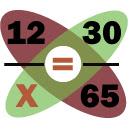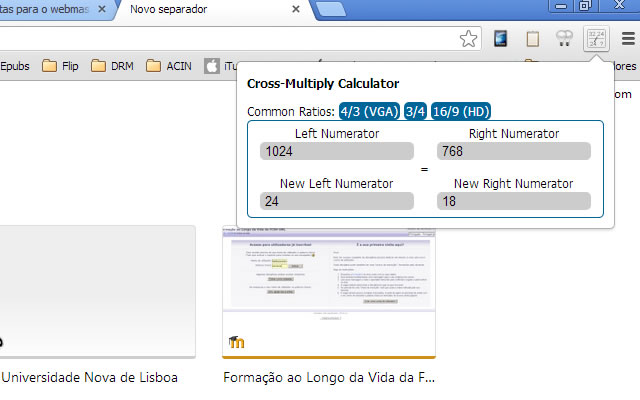# Cross Multiply Calculator

Description from extension meta

## Calculate cross multiply proportions, ratios, sizes,...

Image from storeDescription from store This very simple extension enables you to calculate proportional values, using the cross multiplication rule / vector cross product calculation, also known as the Rule of Three calculation. Can be used to resize images, videos, ... with proportional values.

Installs
54 history
Category
Rating